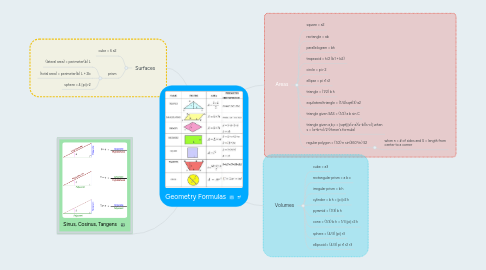# Geometry FormulasVasile Stoica
Get Started. It's FreeGeometry Formulas## 1. Areas

### 1.11. regular polygon = (1/2) n sin(360°/n) S2

1.11.1. when n = # of sides and S = length from center to a corner

## 3. Surfaces

### 3.2. prism

3.2.1. (lateral area) = perimeter(b) L

3.2.2. (total area) = perimeter(b) L + 2b

3.2.3. sphere = 4 [pi] r2